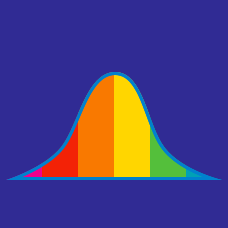Probability

Data - Interquartile Range

What is the upper quartile of the list of positive integers from 1 to 47 inclusive?

What is the upper quartile of the following list of numbers:

$\{ 20, 11, 16, 3, 26, 2, 4 \} ?$

What is the lower quartile of the following list of numbers:

$\{ 1, 15, 8, 5 , 10, 12, 5 \} ?$

What is the interquartile range of the following list of numbers:

$\{ 29, 15, 20, 27, 34, 23, 12\} ?$

What is the interquartile range of the list of positive integers from 1 to 27 inclusive?

×